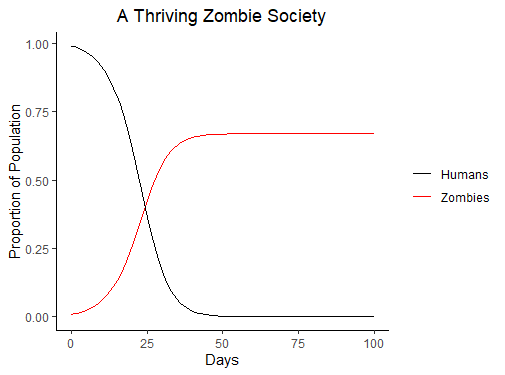# Modelling a Zombie Apocalypse

2020, Mar 28

Recent events have inspired me to model a Zombie apocalypse. In this apocalypse, there is a roving horder of Zombies biting people. If you’re bitten, you can receive treatment and be saved (until the next Zombie takes a bite) or else you turn into a Zombie and contribute to the roving horde, biting people. The model will need a population size ($p$), consisting of a number of Humans ($h$) and Zombies ($z$) on a given day such that

I’ve taken inspiration from the the predator-prey equations to model the number of people bitten on a given day ($b$) with the Zombies’ skill in successfully biting a person ($\beta$) as

We can sense check this equation by setting $p_{day 1} = 1, z_{day 1} = 0.01, \beta = 1$ to get $b_{day 1} = 0.0099$. This makes sense as every Zombie encounter results in a bite but not every Zombie will find a Human, resulting in nearly every Zombie biting a Human.

If bitten, there is a chance ($\theta$) of receiving treatment - perhaps a friend chops off the bitten body part. We can use this to model the growth ($g$) in Zombies on a day as

Setting the chance of receiving treatment ($\theta$) to 1, we see $\theta\beta(p_{day 1} - z_{day 1})z_{day 1}$ exactly matches the number of people bitten and the zombie population doesn’t grow ($g_{day 1} = 0$). If we set $\theta > 1$, more people are treated for bites than receive bites, so let’s not do that.

We now need some way to reduce the number of Zombies. I will model our Humans fighting back, killing Zombies with an effectiveness ($\kappa$) such that the number of Zombies that die in a day ($d$) are

Think of a low $\kappa$ as disorganised gangs with make shift weapons and a high $\kappa$ as a highly organised military effort. We combine everything to get the change in number of Zombies ($\dot{z}$) in a day as

Killing Zombies will decrease our population ($p$) at a rate ($\dot{p}$), so let’s include that in our model

To summarise, we have two equations, one for the spread of Zombies, another for the change in population and we have a restriction on a term

Let’s now think about what this tells us. Whenever $g = d$ the number of Zombies remain constant, that is, when

If the treatment effectiveness ($\theta$) is 1 and the Humans ability kill zombies ($\kappa$) is 0 then Zombie bites become meaningless and neither population changes. Hopefully in this scenario the treatment is more sophisticated than chopping off the bitten body part.

If $g = d$ with $\kappa > 0$, the constant horde of Zombies will sustain itself while the Human population slowly dwindles. I’ve created a chart that starts with 1% of the population as Zombies ($p_{day 1} = 1, z_{day 1} = 0.01$) who can bite 1 Human a day ($\beta = 1$) while the Humans make some effort to save themselves ($\kappa = \theta = 0.5$).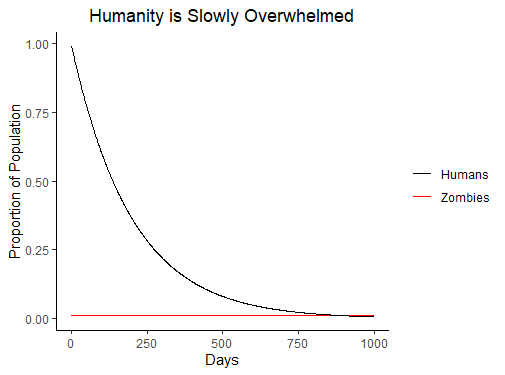Watching the Humans get consumed by a constant number of Zombies isn’t very fun to watch (at least in chart form) so let’s set $g>d$ and watch the Zombie horde grow. I’ll start with Humans who are ok at treating bites but can’t reduce the Zombie population effectively ($\theta = 0.7, \kappa = 0.1$).They quickly get overwhelmed and leave behind a thriving Zombie society with nearly as many Zombies as there were Humans. Perhaps these Zombies form a government, tell horror stories of the Human plague that once ruled the Earth and create mathematical models for infectious diseases.

If the Humans were poor at treating bites, but great Zombie killers ($\theta = 0.1, \kappa = 0.7$) they get overwhelmed a little bit quicker, but the world ends up with fewer Zombies.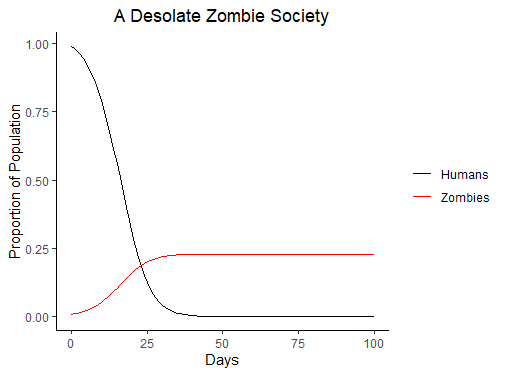Both these scenarios are pretty grim for the Humans. If they could find a way to make it harder for Zombies to reach them by reducing $\beta$ from 1 to 0.5 (keeping $\theta = 0.7, \kappa = 0.1$), the Humans still get overwhelmed but it takes longer. Perhaps the Humans can use the extra time to increase their fighting effectiveness ($\kappa$).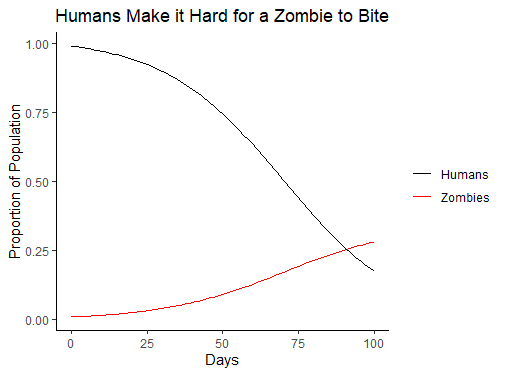At the start of the apocalypse we might have a fixed fighting force and treatment rate, so we can only control the growth of Zombies by instructing people to self isolate barricade themselves in abandoned shopping malls to reduce $\beta$. I’ll plot the effect varying $\beta$ (keeping $\theta = 0.7, \kappa = 0.1$) has on the number of Humans after 50 days.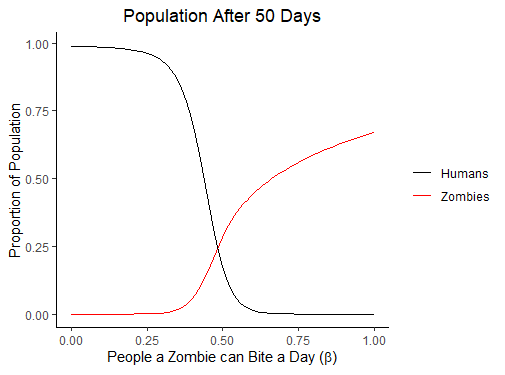When $% $ the Humans will eventually win. For $\beta > \frac{1}{3}$, small differences have a huge impact on the number of Humans left after 50 days. In this Zombie apocalypse, the Humans would be well advised to hide for as long as they can and hope that it’s long enough for the government/post apocalyptic biker gang to form a coherent plan, increasing the fighting capability ($\kappa$) and treatment success ($\theta$). Perhaps they will even hold out long enough for a cure, allowing Zombies to turn back into humans.

Until later, stay healthy.